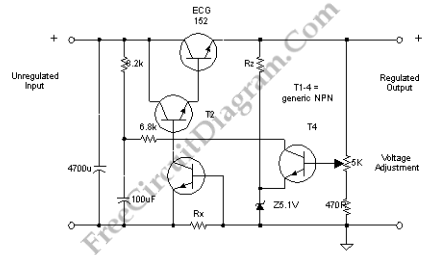# How Discrete Voltage Regulator with Current Limiter WorksThis discrete voltage regulator has complete features that is equivalent with modern voltage regulator integrated circuit (IC). It’s not cheaper if you build discrete one like this, but it’s always interesting to study the schematic diagram to learn how it works. Here is the schematic diagram of the circuit.Volatge Regulation

Look at the 6,8k resistor, it should be dotted at the cross section between transistors. This resistor supply the base current of T2.  The voltage at this base will be followed by the output voltage.  This base voltage will be affected by two other transistor, T4 on the right side and T3 below T2.  At the first time the circuit is powered, T4 and T3 is off, and this base voltage increase. This increasing voltage will appear on the output. At certain point (set by 5K pot and 5.1 zener diode), the voltage output voltage reach the level of T4 activation point, where T4 becomes active to short this T2 base voltage, preventing further increase, and even decrease it if too high. This mechanism is the basic of the regulation.

Current Limiter

What about T3? In normal condition, T3 is always inactive. The only way to get activated is when a voltage is build up across Rx and reach a certain level (Vbe forward bias). Rx is normally a resistor with small value, depends on how much maximum current is allowed for the regulator.  Neglecting the current from resistor 470R and 5.1v Zener diode, almost all current at Rx is coming from the load of this powers supply.  Let say we have T3 with 0,6V Vbe (forward base-emitter bias voltage),  a 0.6Ω Rx resistor will build 0,6 Volts voltage if 1A current is returned from the load, activating T3 to short T2 base voltage, reducing the output voltage to prevent further current increase at the load. This way a 1A current limiter is accomplished if we chose a transistor T3 with 0.6V Vbe and 0.6Ω  resistor for Rx.